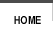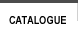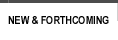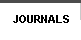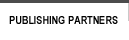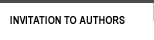sitemap | contact usISBN Author Title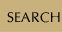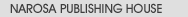Book SeriesJournalsBook Proposal FormWriting a BookUsing Published MaterialRights and PermissionsExamination Copies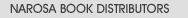List of PublishersBargains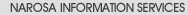Services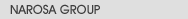About NarosaHistoryMissionGroup CompaniesOur StrengthAlliances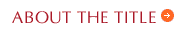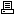view in print mode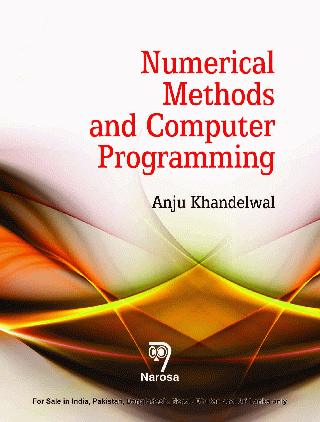Numerical Methods and Computer Programming Author(s): Anju Khandelwal ISBN:    978-81-8487-378-8  E-ISBN:    Publication Year:   2015 Pages:   280 Binding:   Paper Back Dimension:   185mm x 240mm Weight:   457 Textbook

 About the book NUMERICAL METHODS AND COMPUTER PROGRAMMING focuses mainly on numerical method envisaged in almost every field of science and engineering and essentially in any type of work that requires calculations to give precise solutions. The point of numerical analysis is to analyze methods that are used to give approximate number solutions to situations where it is unlikely to find the real solution quickly and to try and improve upon these methods so as to reduce the amount of error generated by computer calculation. The book has embed in its contents the use of computing programming describe algorithmic solutions whose basic ideas are common to a variety of mathematical problems. By means of the methods presented, the reader will acquire the skills besides a fundamental knowledge to successfully work on related subjects in this field. Computing approach enables solution of complex problems with a great number of very simple operations. Table of Contents Preface / Solution of Algebraic and Transcendental Equation: Introduction / Methods for Finding Root of an Equation / Order or Rate of Convergence / Newton-Raphson Method / Method for Complex Root / Lin- Bairstow Method / Graeffs Root Square Method / Comparison / Newton-Raphson Method Program Code in C Language / Newton-Raphson Method MATLAB Code / Graeffes Root Squaring Method Program in C Language / Interpolation and Approximation: Introduction / Lagranges Interpolation Formula / Newton-Divided Difference Formula / Newton Interpolation Formula for Finite Differences / Cubic Spline / Least Squares Approximation using Chebyshev Polynomial / Solution of Linear Simultaneous Equation: Introduction / Choleskys (Crouts) Method / LU Factorization / Iterative Method for Solution of Simultaneous Linear Equation / Numerical Differentiation and Integration: Introduction / Numerical Differentiation / Numerical Integration / Solution of Differential Equation: Introduction / Modified Eulers Method / Runge-Kutta Method of Second Order / Runge-Kutta Method of Third Order / Runge-Kutta Method of Forth Order / Milnes Predictor-Corrector Method / Stability of Ordinary Differential Equation / Index. Audience Undergraduate and Postgraduate Students, Teachers and Researchers

# var message="For Bargains from Narosa Book Distributors, " var neonbasecolor="black" var neontextcolor="red" var neontextcolor2="red" var flashspeed=100 // speed of flashing in milliseconds var flashingletters=2 // number of letters flashing in neontextcolor var flashingletters2=2 // number of letters flashing in neontextcolor2 (0 to disable) var flashpause=0 // the pause between flash-cycles in milliseconds ///No need to edit below this line///// var n=0 if (document.all||document.getElementById){ document.write('<font color="'+neonbasecolor+'" size="5">') for (m=0;m<message.length;m++) document.write('<span id="neonlight'+m+'">'+message.charAt(m)+'</span>') document.write('</font>') } else document.write(message) function crossref(number){ var crossobj=document.all? eval("document.all.neonlight"+number) : document.getElementById("neonlight"+number) return crossobj } function neon(){ //Change all letters to base color if (n==0){ for (m=0;m<message.length;m++) crossref(m).style.color=neonbasecolor } //cycle through and change individual letters to neon color crossref(n).style.color=neontextcolor if (n>flashingletters-1) crossref(n-flashingletters).style.color=neontextcolor2 if (n>(flashingletters+flashingletters2)-1) crossref(n-flashingletters-flashingletters2).style.color=neonbasecolor if (n<message.length-1) n++ else{ n=0 clearInterval(flashing) setTimeout("beginneon()",flashpause) return } } function beginneon(){ if (document.all||document.getElementById) flashing=setInterval("neon()",flashspeed) } beginneon() CLICK HERE

Group
| Companies | Mission | Strength | Values | History | Contact us